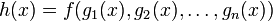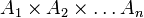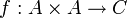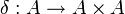# Jointly continuous map

(diff) ← Older revision | Latest revision (diff) | Newer revision → (diff)

## Definition

Suppose$A_1, A_2, \dots, A_n, C$ are topological spaces. A set map$f:A_1 \times A_2 \times \dots \times A_n \to C$ is termed a jointly continuous map if it satisfies the following equivalent conditions:

1.$f$ is a continuous map from$A_1 \times A_2 \times \dots \times A_n$ equipped with the product topology.
2. Suppose$X$ is a topological space and$g_i:X \to A_i$ are all continuous maps. Then, the map$h:X \to C$ given by$h(x) = f(g_1(x),g_2(x),\dots,g_n(x))$ is a continuous map. (This should be true for every choice of$X$ and$g_i$s).

## Relation with separate continuity

Jointly continuous is typically contrasted with the notion of a separately continuous map. A map$f:A_1 \times A_2 \times \dots \times A_n \to C$ is separately continuous if it is continuous from the slice topology on$A_1 \times A_2 \times \dots A_n$. More explicitly, it is continuous in each coordinate fixing the values of all the other coordinates. The slice topology is a finer topology (often, but not necessarily, strictly finer) than the slice topology. Thus, joint continuity is a stronger (and in some cases, strictly stronger) condition than separate continuity.

Joint continuity is the correct condition in most circumstances. For instance, if$f:A \times A \to C$ is separately continuous, we cannot be sure whether$f \circ \delta$ is continuous, where$\delta:A \to A \times A$ is the diagonal embedding. More generally, for a separately continuous map, we cannot guarantee continuity under a simultaneous change of both coordinates.

Thus, all definitions that involve continuity from products use joint continuity. Examples include the definition of topological magma, topological monoid, and topological group, the definition of homotopy between maps, and all the definitions/concepts arising from homotopy.